Courses

SRMJEEE Physics Mock Test - 6

35 Questions MCQ Test SRMJEEE Subject Wise & Full Length Mock Tests | SRMJEEE Physics Mock Test - 6

Description
Attempt SRMJEEE Physics Mock Test - 6 | 35 questions in 50 minutes | Mock test for JEE preparation | Free important questions MCQ to study SRMJEEE Subject Wise & Full Length Mock Tests for JEE Exam | Download free PDF with solutions
QUESTION: 1

Solution:
QUESTION: 2

Solution:
QUESTION: 3

Choke used to limit high frequency A.C. has

Solution:
QUESTION: 4

The angular velocity of the second needle in watch (in rad/s) is

Solution:
QUESTION: 5

When two bodies collide elastically, then

Solution:
QUESTION: 6

An ammeter with internal resistance 90Ω reads 1.85 A when connected in a circuit containing a battery and two resistors 700Ω and 410Ω in series. Actual current will be

Solution:
QUESTION: 7

Cathode rays are

Solution:
QUESTION: 8

The length of iron wire is L and area of cross-section is A. The increase in length is l on applying the force F on its two ends. Which of the statement is correct

Solution:
QUESTION: 9

A gate with only one input and one output is

Solution:
QUESTION: 10

Figure shows a solid hemisphere with a charge of 5nC distributed uniformly through its volume. The hemisphere lies on a plane and point P is located on the plane, along a radial line from the centre of curvature at distance 15cm. The electric potential at point P due to the hemisphere is: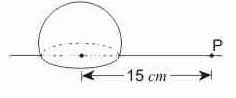Solution:
QUESTION: 11

If the radius of earth's orbit is made 1/4, the duration of an year will become

Solution:
QUESTION: 12

For the moon, the wavelength corresponding to maximum emissive power is 14 μm. The surface temperature of the moon is (Wien's displacement constant = 2.8x10⁻3m−K)

Solution:
QUESTION: 13

A block of mass 10 kg is placed on a rough horizontal surface having coefficient of friction μ = 0.5. If a horizontal force of 100 N is acting on it, then acceleration of the block will be (Take g = 10 m−s⁻2)

Solution:
QUESTION: 14

A flask is filled with 13 gm of an ideal gas at 27°C and its temperture is raised to 52°C. The mass of the gas that has to be released to maintain the temperature of the gas in the flask at 52°C and the pressure remaining the same is

Solution:
QUESTION: 15

A body takes just twice the time as long to slide down a plane inclined at 30° to the horizontal as if the plane were frictionless. The coefficient of friction between the body and the plane is

Solution: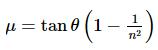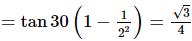QUESTION: 16

A copper wire of resistance 10 Ω is in the form of a perfect circle. Two points A and B on it are connected to a battery of emf 5 V and internal resistance 0.5 Ω. The two segments of the circle have lengths in the ratio 2:3. The net magnetic field induction at the centre of the circle is

Solution:
QUESTION: 17

In a deflection magnetometer experiment the deflections produced separately by two short bar magnets kept at the same distance are 45° and 30° . Then the ratio of the magnetic moments of the two magnets is

Solution:

For deflection magnetometer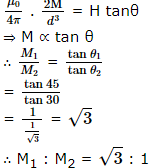QUESTION: 18

A piece of steel floates in mercury. The specific gravities of mercury and steel are 13.6 and 7.8 respectively. For covering the whole piece, some water is poured over the mercury. What part of the steel piece will be inside the mercury?

Solution:

Suppose volume of steel piece is V and
nV is volume of mercury.
nV x 13.6 + (V - nV) x 1 = V x 7.8
n = 0.54

QUESTION: 19

The motion of a particle is given by (a) = t3 − 3t2 + 5, where a is the acceleration in m−s⁻2 and t is the time in seconds. At t = 1 s, velocity of the particle was 6.25 m−s⁻1. The velocity of the particle after 2 seconds will be

Solution:
QUESTION: 20

A particle is moving with a velocity of 5 m−s⁻1 towards east. After 10 s, if its velocity changes to 5 m−s⁻1 towards north, then its acceleration is

Solution:
QUESTION: 21

A body is imparted motion rest to move in a straight line. If it is then obstructed by an opposite force, then

Solution:
QUESTION: 22

If a simple harmonic motion is represented by d2x/dt2 + αx = 0, its time period is

Solution:
QUESTION: 23

Two point particles with masses m₁ and m₂ are thrown at angles θ₁ and θ₂ with the horizontal with speeds of v₁ and v₂ respectively. T, H and R represent the total time of flight, the maximum height attained and the horizontal range covered respectively. If v₁ sin θ₁ = v₂ sin θ₂, then for both the particles

Solution:
QUESTION: 24

A bomber plane is moving horizontally with a speed of 500 ms-1 and a bomb released from it, strikes the ground in 10 s. The angle at which it strikes the ground is (g = 10 m/s2)

Solution:

Here, νx = 500 m/s; νy = gt = 10 x 10 = 100 m/s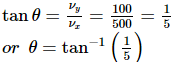QUESTION: 25

A plano convex lens is made refractive index 1.6. The radius of curvature of the curved surface is 60 cm. The focal length of the lens is

Solution:
QUESTION: 26

In an orbital motion, the angular momentum vector is

Solution:
QUESTION: 27

A boat is moving with a velocity 3î+4ĵ with respect to the ground. The water in the river is flowing with a velocity -3î-4ĵ with respect to the ground. The velocity of the boat relative to the water is

Solution:
QUESTION: 28

A liquid wets a solid completely. The meniscus of the liquid in a sufficiently long tube is

Solution:
QUESTION: 29

An electric bulb marked 40 W and 200 V, is used in a circuit of supply voltage 100 V. Now its power is

Solution:
QUESTION: 30

A closed hollow insulated cylinder is filled with gas 0°C and also contains an insulated piston of negligible weight and negligible thickness at the middle point. The gas on one side of the piston is heated to 100°C . If the piston moves 5 cm, the length of the hollow cylinder is

Solution:
QUESTION: 31

Air is pumped into an automobile tyre's tube upto a pressure of 200 kPa in the morning when the air temperature is 22°C During the day temperature rises to 42°C and the tube expands by 2%. The pressure of the air in the tube at this temperature will be approximately

Solution:
QUESTION: 32

The dimensions of Planck's constant and angular momentum are respectively

Solution:
QUESTION: 33

Doppler's effects in sound and light are

Solution:
QUESTION: 34

100 g of a iron ball having velocity 10 m/s collides with a wall at an angle 30° and rebounds with the same angle. If the period of contact between the ball and wall is 1 second, then the force experienced by the wall is

Solution:
QUESTION: 35

A source and listener are both moving towards each other with speed υ/10, where υ is the speed of sound. If the frequency of the note emitted by the source is f, the frequency heard by the listener would be nearly

Solution:Use Code STAYHOME200 and get INR 200 additional OFF Use Coupon Code

Track your progress, build streaks, highlight & save important lessons and more!

Similar ContentRelated tests